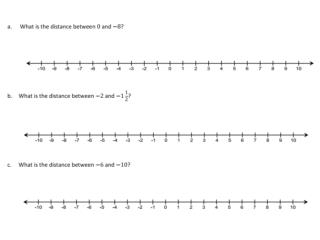DownloadDownload PresentationThe distance between a negative number and a positive number is 12 ½ What are the numbers?

# The distance between a negative number and a positive number is 12 ½ What are the numbers?

Télécharger la présentation## The distance between a negative number and a positive number is 12 ½ What are the numbers?

- - - - - - - - - - - - - - - - - - - - - - - - - - - E N D - - - - - - - - - - - - - - - - - - - - - - - - - - -
##### Presentation Transcript

1. A hiker starts hiking at the beginning of a trail at a point which is 200 feet below sea level. He hikes to a location on the trail that is 580 feet above sea level and stops for lunch. a. What is the vertical distance between 200 feet below sea level and 580 feet above sea level? b. How should we interpret 780 feet in the context of this problem?

2. 2. After lunch, the hiker hiked back down the trail from the point of elevation, which is 580 feet above sea level, to the beginning of the trail which is 200 feet below sea level. a. What is the vertical distance between 580 feet above sea level and 200 feet below sea level? b. What is the change in elevation?

3. The distance between a negative number and a positive number is 12 ½ • What are the numbers?

4. a. Find the distance between −7 and −4. • b. Find the change in temperature if the temperature rises from −18◦F to 15◦ F • c. Would your answer for part (b) be different if the temperature dropped from 15◦F to −18◦ F? • d. Beryl is the first person to finish a 5K race and is standing 15 feet beyond the finish line. Another runner, Jeremy, is currently trying to finish the race and has approximately 14 feet before he reaches the finish line. What is the minimum possible distance between Beryl and Jeremy? • e. What is the change in elevation from 140 feet above sea level to 40 feet below sea level? Explain

5. Using Integer Chips • 5 – 3 • How does this compare to 5 + -3? • 8 - 2

6. -9 - - 5 • What do you notice about your sum as you take negatives away? Is the sum larger or smaller than the starting number? • -6 - - 2

7. 3 - - 5 • 6 - - 4

8. Why does subtracting a negative result in a larger value?

9. -2 – 6 • -3 – 5 • 4 - 9

10. We want to turn all subtraction problems into addition problems • Can you develop rules?